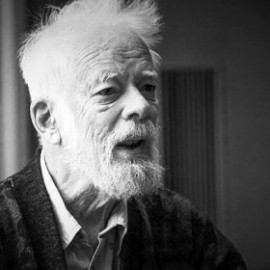Profile

# Edward Nelson

Department of Mathematics, Princeton University, USA
Physics web.math.princeton.edu/~nelson/ math.princeton.eduEdward Nelson (May 4, 1932 – September 10, 2014) was a professor in the Mathematics Department at Princeton University. He was known for his work on mathematical physics and mathematical logic. In mathematical logic, he was noted especially for his internal set theory, and his controversial views on ultrafinitism and the consistency of arithmetic. He also wrote on the relationship between religion and mathematics.
Nelson was born in Decatur, Georgia. He received his Ph.D. in 1955 from the University of Chicago, where he worked with Irving Segal. He was a member of the Institute for Advanced Study from 1956 to 1959. He held a position at Princeton University starting in 1959, attaining the rank of professor there in 1964 and retiring in 2013.

In 2012 he became a fellow of the American Mathematical Society. He died in Princeton, New Jersey on September 10, 2014.

Nelson made contributions to the theory of infinite-dimensional group representations, the mathematical treatment of quantum field theory, the use of stochastic processes in quantum mechanics, and the reformulation of probability theory in terms of non-standard analysis.

For many years he worked on mathematical physics and probability theory, and retained a residual interest in these fields, particularly in possible extensions of stochastic mechanics to field theory.

In 1950, Nelson formulated a popular variant of the four color problem. What is the chromatic number, denoted \chi, of the plane? In more detail, what is the smallest number of colors sufficient for coloring the points of the Euclidean plane in such a way that no two points of the same color are unit distance apart? We know by simple arguments that 4 ≤ χ ≤ 7. The problem was introduced to a wide mathematical audience by Martin Gardner in his October 1960 Mathematical Games column. The chromatic number problem, also now known as the Hadwiger–Nelson problem, was also a favorite of Paul Erdős, who mentioned it frequently in his problems lectures.
In the later part of his career, he worked on mathematical logic and the foundations of mathematics. One of his goals was to extend IST (Internal Set Theory—a version of a portion of Abraham Robinson's non-standard analysis) in a natural way to include external functions and sets, in a way that provides an external function with specified properties unless there is a finitary obstacle to its existence. Other work centered on fragments of arithmetic, studying the divide between those theories interpretable in Raphael Robinson's Arithmetic and those that are not; computational complexity, including the problem of whether P is equal to NP or not; and automated proof checking.

In September 2011, Nelson announced that he had proved that Peano arithmetic was logically inconsistent. An error was found in the proof, and he retracted the claim.